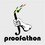# Proofathon: This Weekend

Hello fellow mathematicians,

This upcoming weekend marks the inaugural Proofathon contest. Essentially, Proofathon is a USAMO-like, online math contest administered over the course of two days, eight problems. Show your support and compete in the inaugural Proofathon! Also, note that if we get over 200 likes on our Facebook page, we will have a special surprise.

Until then, I'll leave you with one of our shortlisted problems:

Show that none of the elements in the set $\{49,409,4009,\dots\}$ is a perfect cube.Note by Cody Johnson
6 years, 8 months ago

This discussion board is a place to discuss our Daily Challenges and the math and science related to those challenges. Explanations are more than just a solution — they should explain the steps and thinking strategies that you used to obtain the solution. Comments should further the discussion of math and science.

When posting on Brilliant:

• Use the emojis to react to an explanation, whether you're congratulating a job well done , or just really confused .
• Ask specific questions about the challenge or the steps in somebody's explanation. Well-posed questions can add a lot to the discussion, but posting "I don't understand!" doesn't help anyone.
• Try to contribute something new to the discussion, whether it is an extension, generalization or other idea related to the challenge.

MarkdownAppears as
*italics* or _italics_ italics
**bold** or __bold__ bold
- bulleted- list
• bulleted
• list
1. numbered2. list
1. numbered
2. list
Note: you must add a full line of space before and after lists for them to show up correctly
paragraph 1paragraph 2

paragraph 1

paragraph 2

[example link](https://brilliant.org)example link
> This is a quote
This is a quote
    # I indented these lines
# 4 spaces, and now they show
# up as a code block.

print "hello world"
# I indented these lines
# 4 spaces, and now they show
# up as a code block.

print "hello world"
MathAppears as
Remember to wrap math in $$ ... $$ or $ ... $ to ensure proper formatting.
2 \times 3 $2 \times 3$
2^{34} $2^{34}$
a_{i-1} $a_{i-1}$
\frac{2}{3} $\frac{2}{3}$
\sqrt{2} $\sqrt{2}$
\sum_{i=1}^3 $\sum_{i=1}^3$
\sin \theta $\sin \theta$
\boxed{123} $\boxed{123}$

Sort by:

Solution: Cubes are always $-1,0,1\pmod 9$, and since the given numbers are all $4\pmod 9$, there are no cubes in the set. $\blacksquare$

- 6 years, 8 months ago

How many perfect fifth powers can be written in the form of $9\cdot2^k-4$?

- 6 years, 8 months ago

Let $m^5=9\cdot 2^k+6$. From this, we see that $3|m^5\implies 3|m$, and so $9|m^5$. However, $9\cdot 2^k+6\equiv 6\pmod 9$, a contradiction. Therefore, the answer is $\boxed{0}$.

This can also be found by writing out the quintic residues modulo 9.

- 6 years, 8 months ago

I messed up (addition error): $9\cdot2^k-4$ Sorry!

- 6 years, 8 months ago

Let $m^5=9\cdot 2^k-4$.

If $k<5$, then we can check manually: \begin{aligned} &k=0\implies m^5=5\text{, no solution} \\ &k=1\implies m^5=14\text{, no solution} \\ &k=2\implies m^5=32,\ m=2 \\ &k=3\implies m^5=68\text{, no solution} \\ &k=4\implies m^5=140\text{, no solution} \end{aligned}

Otherwise, if $k>4$, then $2|9\cdot 2^k-4\implies 2|m$. Then, $32|9\cdot 2^k-4$. Hence, $32|9\cdot 32\cdot 2^{k-5}-4$, which is not possible.

Therefore, the answer is $\boxed{1}$. $\blacksquare$

- 6 years, 8 months ago

I did modulo 8 with three cases, but that works as well! Good job!

- 6 years, 8 months ago

You can always search for Proofathon in facebook :) P.S.-The link is working fine for me.

- 6 years, 8 months ago

Is there some easy way to figure out that the numbers are all congruent to $4 \pmod 9$? This is how I figured it:

Considered modulo 9, the terms are $4*10^k$ for all $k \geq 1$. It can be shown that the numbers $36, 396, 3996, 39996, 399....96$ are all multiples of $9$, namely they are $9 * 44...4$, so thus the numbers $40, 400, 4000, 40000...$ are congruent to $4 \pmod 9$.

- 6 years, 8 months ago

More simply, $4\cdot10^k+9\equiv4\cdot1^k+9\equiv\boxed{4}\pmod{9}$

- 6 years, 8 months ago

Let $S(n)$ denote the sum of the digits of $n$. Then, $n\equiv S(n)\pmod 9$ (this is not hard to prove).

- 6 years, 8 months ago

If anything, look at our logo!

- 6 years, 8 months ago

how do I submit the problems??is there any registration??

- 6 years, 8 months ago

If you want to submit original problems for consideration for use on future contests, you can email us at Proofathon@gmail.com. If you are asking where to submit solutions to the problems for the contests, visit our contest page http://proofathon.org/Pages/contests.php on the date of the contest. The first contest will be held this weekend from October 26 at 12:00 AM EDT to October 27 at 11:59 EDT.

To register, simply create an account at http://proofathon.org/Pages/account.php and log on with that account on the contest date.

- 6 years, 8 months ago

Note that the date has changed, and Proofathon is starting this Friday at midnight (which is in 4 hours).

- 6 years, 8 months ago

The contest is ongoing. One can find the problems here.

- 6 years, 8 months ago